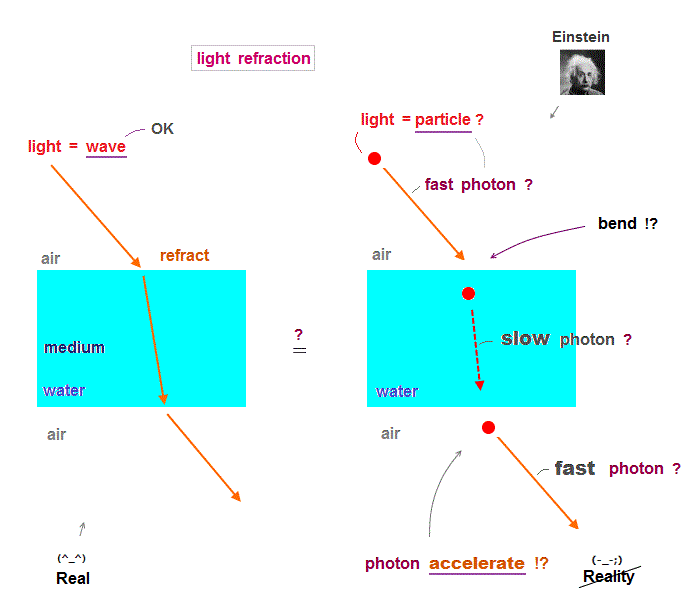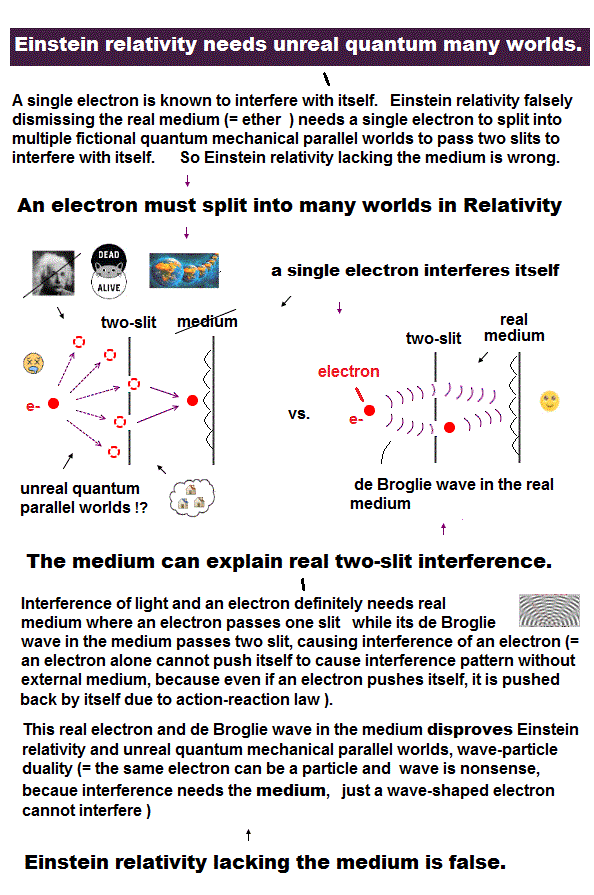﻿ Virtual photon by unreal quantum mechanics

# Virtual photon

## Energy momentum  must be conserved.

### [ Total energy and momentum must be always conserved. ]

(Fig.1)  ↓ When an electron 1 hits electron 2 at rest, conservation holds.Total energy and momentum must be always conserved in any reactions.

An electron 1 moving at speed v collides with a stationary electron 2.
The electron 2 starts to move at v instead of the electron 1.

Of course, this case conserves total energy and momentum.

## Photon = light particle  violates basic law.

### [ If an electron emits a photon, energy-momentum is NOT conserved ! ]

(Fig.2)  Because the mass of a photon (= light ? ) is different from electron.Quantum mechanics claims that each electron can emit or absorb a photon (= light particle ? ), when its energy changes.

But in this case, total energy and momentum are NOT conserved !
The masses of an electron and a photon (= zero ) are different.

So the ratios of energy to momentum are different in an electron and a photon !

To conserve energy and momentum, the photon emitted from an electron must be virtual (= NOT real ) particle.

## Virtual photon  has  unreal mass.

### [ Energy-momentum conserved → unreal virtual photon ! ]

(Fig.3)  Mass squared of virtual photon is negative ( m2 < 0 ) !?For a photon emitted from an electron to conserve total energy and momentum, it must be virtual photon with unreal mass.

This virtual photon mass is not zero, which contradicts the real photon.

The mass squared of the virtual photon is negative, which is the reason why it's called "virtual" ( this p.3 ).

As you see, the photon by quantum mechanics violates the basic physical law, so wrong.

## When energy momentum is conserved ..

### [ If an electron emits a photon, conserving energy momentum .. ]

(Fig.4)  Energy (= E)   momentum (= p )  are conserved.When an moving electron (= energy E, momentum p ) stops after emitting a photon, its mometum is zero ( p = 0 ) and its energy is rest mass ( E = mc2 ).

The emitted photon gains the energy and momentum equal to the difference between moving and stationary electron.

## Mass squared of virtual photon is negative.

### [ Energy-momentum conserved → Einstein equation → unreal photon ]

(Fig.5)  The square of photon's mass becomes negative ( m2 < 0 ) !?Suppose total energy and momentum are conserved when an electron emits a photon, insert these values into Einstein equation.

If this photon is real, its mass must be zero.
But the mass of virtual photon is NOT zero.

The mass squared of emitted photon is always negative ( m2 < 0 ) = unreal photon !

So the quantum mechanics's photon model violates basic phisycal law.  We have to abandon this quantum mechanics based on unreal virtual photon.

## Photon model  collapsed.

### [ We must introduce "real medium" in an electron emitting light. ]

(Fig.6)  Electron emits light = causes light wave in surrounding medium.If an electron emits a photon (= light ? ) and transfers its kinetic energy and momentum to newly emerging photon, it becomes unreal virtual photon.

Because the ratio of energy to momentum are different between an electron and a photon with different masses.

To solve this problem, we have to rely on other material which already exists as "surrounding medium" around an electron.

When the total energy of a electron and a proton changes, it causes light wave carrying that amount of energy in surrounding medium.

This model of light wave in the medium does not violate energy-momentum conservation law without causing virtual photon carrying unreal mass.

## Light = photon  is stupid.

### [ Light particle decelerates → accelerates between media ? ]

(Fig.7)  Light photon particle  fast → slow → fast  again !?We know the light becomes slower and is bent, when it enters water from air.

And when light goes out of water, it accelerates and is bent back again ?

If light is a photon particle, the bending angle is random because of scattering.  But refractive angle is fixed in each medium.

And this photon particle must be accelerated again when it exits water.  There is NO strange thing to reaccelerate light in water !

Light refraction needs the concept of "medium" transmitting light !

### [ Special relativity contradicts de Broglie theory. ]

(Fig.8)  Observer moves → interference disappears from screen ?The most fatal flaw in Einstein special relativity is that it contradicts de Broglie theory.

de Broglie theory says the electron wavelength depends on its velocity, and can be confirmed in various experiments such as double-slit.

Seen by stationary observer (= K ), the electron is moving at v and generates its de Broglie wave causing interference pattern on the screen.

But seen from moving observer (= K' ), the electron appears to stop, so it generates neither de Broglie wave nor interference on the screen !

This is clearly a paradox, and shows that Einstein relativity and mass are wrong.

## Two-slit experiment needs "medium".

### [ A electron splits into parallel worlds in two-slit ? ]

(Fig.9)  A single electron interferes with each other.In two-slit experiment, even a single electron can interfere with each other, meaning a electron splits into two different paths ?

Quantum mechanics claims that an electron splits into two different parallel universe, and it shows fantasy multiverse = many worlds.

Since Einstein rejected 'real medium', physicists have to rely on unreal virtual particle and parallel worlds in actual experiments.

If we admit 'real medium' in space, we can explain a single particle's interference without fantasy parallel universes and Einstein paradox.

Top journals spreading an unreal cat = dead and alive at once is greedy university's political tool to criticize government and Brexit.

Fake news media also deceive people by fictional parallel universe.  Unreal many-worlds has nothing to do with biomedical !

Quantum world is useless except for university's lecture, video, twitter.

All science fields including biomedical stop at useless basic physics !2017/6/23 updated. Feel free to link to this site.